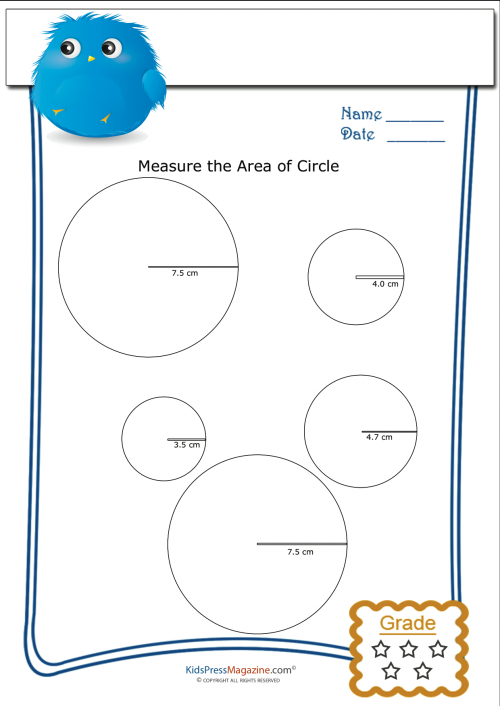# Measuring Area Worksheet – Circles 4

The area of a circle is equal to Pi (3.14) multiplied by the circle’s radius squared (or radius multiplied by radius), or A = x r 2

Teaching this formula to your children will help them succeed in geometry!